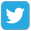Prepare forOlympiad Redeem Vouchers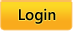Common Core:  MATH |  LANGUAGE ARTS |  SCIENCE
 Grades: Kindergarten |  First |  Second |  Third |  Fourth |  Fifth

# Common Core

Skills available for Common Core third-grade math standards

Click on the name of a skill to practice that skill.

### Operations and Algebraic Thinking.

Represent and solve problems involving multiplication and division.
• CC.3.OA.1 Interpret products of whole numbers, e.g., interpret 5 × 7 as the total number of objects in 5 groups of 7 objects each. For example, describe a context in which a total number of objects can be expressed as 5 × 7.

• CC.3.OA.2 Interpret whole-number quotients of whole numbers, e.g., interpret 56 ÷ 8 as the number of objects in each share when 56 objects are partitioned equally into 8 shares, or as a number of shares when 56 objects are partitioned into equal shares of 8 objects each. For example, describe a context in which a number of shares or a number of groups can be expressed as 56 ÷ 8.

• CC.3.OA.3 Use multiplication and division within 100 to solve word problems in situations involving equal groups, arrays, and measurement quantities, e.g., by using drawings and equations with a symbol for the unknown number to represent the problem.

• CC.3.OA.4 Determine the unknown whole number in a multiplication or division equation relating three whole numbers. For example, determine the unknown number that makes the equation true in each of the equations 8 × ? = 48, 5 = __÷ 3, 6 × 6 = ?.

Understand properties of multiplication and the relationship between multiplication and division.
• CC.3.OA.5 Apply properties of operations as strategies to multiply and divide. Examples: If 6 × 4 = 24 is known, then 4 × 6 = 24 is also known. (Commutative property of multiplication.) 3 × 5 × 2 can be found by 3 × 5 = 15 then 15 × 2 = 30, or by 5 × 2 = 10 then 3 × 10 = 30. (Associative property of multiplication.) Knowing that 8 × 5 = 40 and 8 × 2 = 16, one can find 8 × 7 as 8 × (5 + 2) = (8 × 5) + (8 × 2) = 40 + 16 = 56. (Distributive property.) (Students need not use formal terms for these properties.)

• CC.3.OA.6 Understand properties of multiplication and the relationship between multiplication and division. Understand division as an unknown-factor problem. For example, divide 32 ÷ 8 by finding the number that makes 32 when multiplied by 8.

Multiply and divide within 100
• CC.3.OA.7 Fluently multiply and divide within 100, using strategies such as the relationship between multiplication and division (e.g., knowing that 8 × 5 = 40, one knows 40 ÷ 5 = 8) or properties of operations. By the end of Grade 3, know from memory all products of one-digit numbers.

Solve problems involving the four operations, and identify and explain patterns in arithmetic.
• CC.3.OA.8 Solve two-step word problems using the four operations. Represent these problems using equations with a letter standing for the unknown quantity. Assess the reasonableness of answers using mental computation and estimation strategies including rounding. (This standard is limited to problems posed with whole numbers and having whole-number answers; students should know how to perform operations in the conventional order when there are no parentheses to specify a particular order (Order of Operations).)

• CC.3.OA.9 Identify arithmetic patterns (including patterns in the addition table or multiplication table), and explain them using properties of operations. For example, observe that 4 times a number is always even, and explain why 4 times a number can be decomposed into two equal addends.

### Number and Operations in Base Ten

Use place value understanding and properties of operations to perform multi-digit arithmetic.
• CC.3.NBT.1 Use place value understanding to round whole numbers to the nearest 10 or 100.

• CC.3.NBT.2 Fluently add and subtract within 1000 using strategies and algorithms based on place value, properties of operations, and/or the relationship between addition and subtraction. (A range of algorithms may be used.)

• CC.3.NBT.3 Multiply one-digit whole numbers by multiples of 10 in the range 10-90 (e.g., 9 × 80, 5 × 60) using strategies based on place value and properties of operations. (A range of algorithms may be used.)

### Number and Operations—Fractions

Develop understanding of fractions as numbers.
• CC.3.NF.1 Understand a fraction 1/b as the quantity formed by 1 part when a whole is partitioned into b equal parts; understand a fraction a/b as the quantity formed by a parts of size 1/b. (Grade 3 expectations in this domain are limited to fractions with denominators 2, 3, 4, 6, and 8.)

• CC.3.NF.2 Understand a fraction as a number on the number line; represent fractions on a number line diagram. (Grade 3 expectations in this domain are limited to fractions with denominators 2, 3, 4, 6, and 8.)
• CC.3.NF.2a Represent a fraction 1/b on a number line diagram by defining the interval from 0 to 1 as the whole and partitioning it into b equal parts. Recognize that each part has size 1/b and that the endpoint of the part based at 0 locates the number 1/b on the number line. (Grade 3 expectations in this domain are limited to fractions with denominators 2, 3, 4, 6, and 8.)
• CC.3.NF.2b Represent a fraction a/b on a number line diagram by marking off a lengths 1/b from 0. Recognize that the resulting interval has size a/b and that its endpoint locates the number a/b on the number line. (Grade 3 expectations in this domain are limited to fractions with denominators 2, 3, 4, 6, and 8.)

Develop understanding of fractions as numbers.
• CC.3.NF.3 Explain equivalence of fractions in special cases, and compare fractions by reasoning about their size. (Grade 3 expectations in this domain are limited to fractions with denominators 2, 3, 4, 6, and 8.)

• CC.3.NF.3a Understand two fractions as equivalent (equal) if they are the same size, or the same point on a number line. (Grade 3 expectations in this domain are limited to fractions with denominators 2, 3, 4, 6, and 8.)
• CC.3.NF.3b Recognize and generate simple equivalent fractions (e.g., 1/2 = 2/4, 4/6 = 2/3), Explain why the fractions are equivalent, e.g., by using a visual fraction model. (Grade 3 expectations in this domain are limited to fractions with denominators 2, 3, 4, 6, and 8.)

• CC.3.NF.3c Express whole numbers as fractions, and recognize fractions that are equivalent to whole numbers. Examples: Express 3 in the form 3 = 3/1; recognize that 6/1 = 6; locate 4/4 and 1 at the same point of a number line diagram. (Grade 3 expectations in this domain are limited to fractions with denominators 2, 3, 4, 6, and 8.)

• CC.3.NF.3d Compare two fractions with the same numerator or the same denominator, by reasoning about their size, Recognize that valid comparisons rely on the two fractions referring to the same whole. Record the results of comparisons with the symbols >, =, or <, and justify the conclusions, e.g., by using a visual fraction model. (Grade 3 expectations in this domain are limited to fractions with denominators 2, 3, 4, 6, and 8.)

### Measurement and Data

Solve problems involving measurement and estimation of intervals of time, liquid volumes, and masses of objects
• CC.3.MD.1 Tell and write time to the nearest minute and measure time intervals in minutes. Solve word problems involving addition and subtraction of time intervals in minutes, e.g., by representing the problem on a number line diagram.

• CC.3.MD.2 Measure and estimate liquid volumes and masses of objects using standard units of grams (g), kilograms (kg), and liters (l). (Excludes compound units such as cm^3 and finding the geometric volume of a container.) Add, subtract, multiply, or divide to solve one-step word problems involving masses or volumes that are given in the same units, e.g., by using drawings (such as a beaker with a measurement scale) to represent the problem. (Excludes multiplicative comparison problems (problems involving notions of “times as much.”)

Represent and interpret data.
• CC.3.MD.3 Draw a scaled picture graph and a scaled bar graph to represent a data set with several categories. Solve one- and two-step “how many more” and “how many less” problems using information presented in scaled bar graphs. For example, draw a bar graph in which each square in the bar graph might represent 5 pets.
• CC.3.MD.4 Generate measurement data by measuring lengths using rulers marked with halves and fourths of an inch. Show the data by making a line plot, where the horizontal scale is marked off in appropriate units—whole numbers, halves, or quarters.

Geometric measurement: understand concepts of area and relate area to multiplication and to addition.
• CC.3.MD.5 Recognize area as an attribute of plane figures and understand concepts of area measurement. -- a. A square with side length 1 unit, called “a unit square,” is said to have “one square unit” of area, and can be used to measure area. -- b. A plane figure which can be covered without gaps or overlaps by n unit squares is said to have an area of n square units.

• CC.3.MD.6 Measure areas by counting unit squares (square cm, square m, square in, square ft, and improvised units).

• CC.3.MD.7 Relate area to the operations of multiplication and addition.
• CC.3.MD.7a Find the area of a rectangle with whole-number side lengths by tiling it, and show that the area is the same as would be found by multiplying the side lengths.
• CC.3.MD.7b Multiply side lengths to find areas of rectangles with whole-number side lengths in the context of solving real world and mathematical problems, and represent whole-number products as rectangular areas in mathematical reasoning.
• CC.3.MD.7c Use tiling to show in a concrete case that the area of a rectangle with whole-number side lengths a and b + c is the sum of a × b and a × c. Use area models to represent the distributive property in mathematical reasoning.
• CC.3.MD.7d Recognize area as additive. Find areas of rectilinear figures by decomposing them into non-overlapping rectangles and adding the areas of the non-overlapping parts, applying this technique to solve real world problems.

Geometric measurement: recognize perimeter as an attribute of plane figures and distinguish between linear and area measures.
• CC.3.MD.8 Solve real world and mathematical problems involving perimeters of polygons, including finding the perimeter given the side lengths, finding an unknown side length, and exhibiting rectangles with the same perimeter and different area or with the same area and different perimeter.

### Geometry

Reason with shapes and their attributes.
• CC.3.G.1 Understand that shapes in different categories (e.g., rhombuses, rectangles, and others) may share attributes (e.g., having four sides), and that the shared attributes can define a larger category (e.g., quadrilaterals). Recognize rhombuses, rectangles, and squares as examples of quadrilaterals, and draw examples of quadrilaterals that do not belong to any of these subcategories.

• CC.3.G.2 Partition shapes into parts with equal areas. Express the area of each part as a unit fraction of the whole. For example, partition a shape into 4 parts with equal area, and describe the area of each part is 1/4 of the area of the shape.

 Connect and Follow About Intelliseeds Learning My Account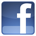Facebook Benefits Membership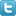Twitter Plans and Pricing Skills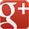Google+ FAQ Wall of Fame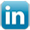LinkedIn Newsletter Student Reports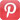Pinterest Careers Rewards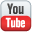Youtube Contact Us What is IntelliAbility?Our Partners Terms & Conditions Tell a FriendNon Profit Partners Testimonials User Guide PTA Fundraising Offers Common Core Math Schools Common Core ELA Virginia SOL Content Team
 Welcome to Intelliseeds Learning Smart learning for your child Intelliseeds learning provides practice in several subjects including Math, Reading Comprehension, Language Arts, Logical Reasoning, Mental Ability, Analytical Ability and Critical Thinking, which are essential to the success of every student. Skills are organized by grades, topics, practice tests and time bound tests. The Math section covers skills such as counting, number operations, algebra, story problems and many more. The IntelliAbility section focuses on thinking skills, patterns, sequences, problem analysis, analogies and over 50 other topics to help develop your child's brain. Click here to learn more about IntelliAbility. The Language Arts section includes reading comprehension, nouns, verbs, adverbs, adjectives, predicates, vowel sounds and many more grammar skills.Privacy | © 2022 Intelliseeds Learning™